#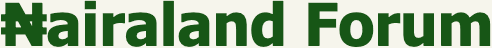Welcome, Guest: Join Nairaland / LOGIN! / Trending / Recent / New
Stats: 2,755,086 members, 6,544,976 topics. Date: Tuesday, 19 October 2021 at 04:49 AM

## I.Q TEST: Let's See How Sound You Are In Quantitative Reasoning - Family - Nairaland

(1) (2) (3) (4) (5) (Reply) (Go Down)

 I.Q TEST: Let's See How Sound You Are In Quantitative Reasoning by matrix199(m): 9:47am On Apr 12, 2020 I.Q challenge. Let's see how sound you are in quantitative reasoning. Here are two I.Q Trivia Puzzles......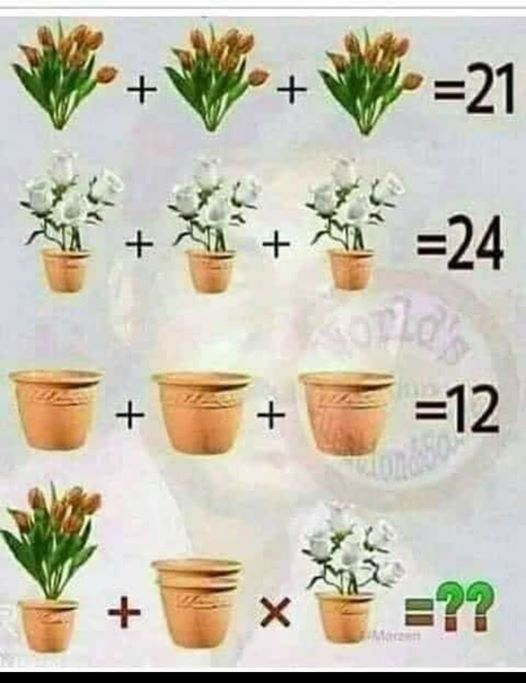Re: I.Q TEST: Let's See How Sound You Are In Quantitative Reasoning by matrix199(m): 9:52am On Apr 12, 2020 I'll reveal the answers to pix 1 and pix 2 and acknowledge those who got it right later on. cc: RoyalRoyModified: I'm a very discreet person. The term 'acknowledge' means more than you might think. You never can tell! Puzzle 1For picture 1; let x represents the helmet. It implies that x + x + x = 30 3x = 30 x = 30/3 = 10 so x is 10For picture 2, let y represents the car. It implies that y + y+ x = 20 since x is 10 y + y + 10 = 20 2y + 10 = 20 2y = 20 - 10 2y = 10 y = 10/2 = 5 so y is 5For picture 3, let z represents the pair of trophies( 2 trophies). It implies that z + z + y = 13 since y is 5 z + z + 5 = 13 2z + 5 = 13 2z = 13 - 5 2z = 8 z = 8/2 = 4 so z is 4 But remember that z is a pair of trophies It implies that 1 trophy is 4/2 = 2For Picture 4, x(10) + y(5+10) x 1/2 of z(2) _____ 10 + 5 x 2Apply BODMAS _______________ 10 + (15 x 2) 10 + 30 = 40. Puzzle 2Take a good look at each quantitative picture. The 1st picture clearly shows 7 petals in each flower pot(7 + 7 + 7 = 21), discreetly indicating that each petal is 1. The 2nd picture also shows 4 roses in each flower pot. it implies that ( 4x2 + 4x2 + 4x2 =8 + 8 + 8= 24 or 12x2 = 24). The 3rd picture indicates that each empty pot is 4, that is ( 4 + 4 + 4 = 12). Now, the 4th picture shows a flower pot of 6 petals( each petal is 1, totalling 6 in combined value) + (double empty flower pots having a value of 4+4=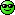x (a flower pot of 5 roses having a combined value of 10(since each rose is 2 in value) That is, 6 + 8 x 10 Don't forget to apply "BODMAS" 6 + (8 x 10) 6 + 80 = 86. Thus, for puzzle 1, the answer is 40! for puzzle 2, the answer is 86! Re: I.Q TEST: Let's See How Sound You Are In Quantitative Reasoning by Joelbm631(m): 9:53am On Apr 12, 2020 6088 2 Likes Re: I.Q TEST: Let's See How Sound You Are In Quantitative Reasoning by Realcash224(m): 10:09am On Apr 12, 2020 first puzzles = 30 Second puzzles = 184 Re: I.Q TEST: Let's See How Sound You Are In Quantitative Reasoning by Spechialone(f): 10:14am On Apr 12, 2020 4075 1 Like Re: I.Q TEST: Let's See How Sound You Are In Quantitative Reasoning by emmflexcsc400(m): 10:28am On Apr 12, 2020 3039 1 Like Re: I.Q TEST: Let's See How Sound You Are In Quantitative Reasoning by Rebelutionary: 10:30am On Apr 12, 2020 30 88 Re: I.Q TEST: Let's See How Sound You Are In Quantitative Reasoning by ADUKKY(f): 10:31am On Apr 12, 2020 Puzzle 1 = 40 Puzzle 2= 82 Re: I.Q TEST: Let's See How Sound You Are In Quantitative Reasoning by sisisioge: 10:32am On Apr 12, 2020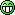19 , 19. 1 Like Re: I.Q TEST: Let's See How Sound You Are In Quantitative Reasoning by Heiterkeit(f): 10:33am On Apr 12, 2020 19 each for both questions. 1 Like Re: I.Q TEST: Let's See How Sound You Are In Quantitative Reasoning by Somzee(f): 10:39am On Apr 12, 2020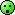1 Like 1 Share Re: I.Q TEST: Let's See How Sound You Are In Quantitative Reasoning by Epositive(m): 11:47am On Apr 12, 2020 1.202.82cc: Martinez19 1 Like Re: I.Q TEST: Let's See How Sound You Are In Quantitative Reasoning by SmileDance(f): 12:01pm On Apr 12, 2020 3039 Re: I.Q TEST: Let's See How Sound You Are In Quantitative Reasoning by ibkayee(f): 12:16pm On Apr 12, 2020 1. 402. 82 Re: I.Q TEST: Let's See How Sound You Are In Quantitative Reasoning by UmmuFarrah(f): 12:21pm On Apr 12, 2020 1. 20 2. 82 Re: I.Q TEST: Let's See How Sound You Are In Quantitative Reasoning by nautybride: 1:03pm On Apr 12, 2020 1. 202. 75 Re: I.Q TEST: Let's See How Sound You Are In Quantitative Reasoning by Katier00(f): 1:04pm On Apr 12, 2020 3088 Re: I.Q TEST: Let's See How Sound You Are In Quantitative Reasoning by Hallenjay: 1:13pm On Apr 12, 2020 25 for d first upload 82 for d second puzzle Re: I.Q TEST: Let's See How Sound You Are In Quantitative Reasoning by immaq8(f): 1:19pm On Apr 12, 2020 1. 172. 152 Re: I.Q TEST: Let's See How Sound You Are In Quantitative Reasoning by Mechatronika(m): 1:25pm On Apr 12, 2020 1. 402. 75 1 Like Re: I.Q TEST: Let's See How Sound You Are In Quantitative Reasoning by yellowgarri2(m): 1:41pm On Apr 12, 2020 1. 20 2 Likes Re: I.Q TEST: Let's See How Sound You Are In Quantitative Reasoning by Nekky5(f): 1:46pm On Apr 12, 2020 1. 602. 88 3 Likes Re: I.Q TEST: Let's See How Sound You Are In Quantitative Reasoning by Tocynone(m): 1:47pm On Apr 12, 2020 1- 202-75 Re: I.Q TEST: Let's See How Sound You Are In Quantitative Reasoning by Nkemella: 1:48pm On Apr 12, 2020 The first puzzle the answer is 30.While the second one is 120. Re: I.Q TEST: Let's See How Sound You Are In Quantitative Reasoning by olaoyekemi(f): 2:07pm On Apr 12, 2020 1. 302. 75 Re: I.Q TEST: Let's See How Sound You Are In Quantitative Reasoning by Blackbelly(m): 2:11pm On Apr 12, 2020 There's an unidentified variable in both puzzles. It is not indicated if the variable is based on addition or multiplication. From algebra, we know that a×b=ab therefore, I'll assume that the combination of unlike terms was made by multiplication. If so, the first puzzle will be mathematically expressed as:10+(10×5)×210+50×2 Using the rule of BODMAS,10+100=110For the 2nd puzzle, the mathematical expression goes thus:(7×4)+(4+4)×828+8×8Using the same BODMAS rule:28+64=92In summary:Puzzle 1 is 110Puzzle 2 is 92Modified: OP in your answer to the second puzzle, you didn't take into account that the flowers are in a pot, you only calculated for the flowers. Secondly there is no visual clue to prove that the pot in the middle has two rings. It still appears to be two pots.I also don't agree with your answer that the little helmet on the middle racecar was to misdirect the observer. An ideal puzzle always takes into little details into account. If we suggest that some obvious visual clues are meant to throw off the observer, we might as well come to a conclusion that the designer of the puzzle is the only one capable of answering the puzzle as it has already defied basic logic.I would suggest you screenshot the correct answer as solved by the puzzle designer, that way we can be rest assured of its validity, because what you put up there is your own opinion as with all of us.Nevertheless, I'll put this up on Quora. Hopefully, I'll be able to trace the original answer.Çiao Re: I.Q TEST: Let's See How Sound You Are In Quantitative Reasoning by Khemhyte(f): 2:24pm On Apr 12, 2020 Pix 1 = 30Pix 2 = 152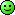Re: I.Q TEST: Let's See How Sound You Are In Quantitative Reasoning by Taymi: 2:27pm On Apr 12, 2020 1- 402- 82 Re: I.Q TEST: Let's See How Sound You Are In Quantitative Reasoning by GraciousGiver: 2:40pm On Apr 12, 2020 1. 10+15*4 equals 100. 2. 11+2(4)*8 equals 152. Re: I.Q TEST: Let's See How Sound You Are In Quantitative Reasoning by Richy4(m): 2:56pm On Apr 12, 2020 First puzzle Blue stuffs = 10 each Red cars = 5 each Yellow cup =4 per setTherefore 10 + 5 × 2 = 20Second puzzle First green plant = 7 eachWhite flowers = 8 each Flower pots = 4 each Therefore 7 +8 × 8 = 71Modified : (just noticed that in that pic one cup only was represented which means each set 4/2 =2Two flower pots stuck on that pic. So each flower pots 4. So 4+4 =8 Re: I.Q TEST: Let's See How Sound You Are In Quantitative Reasoning by sisisioge: 2:58pm On Apr 12, 2020 Richy4:First puzzle Blue stuffs = 10 each Red cars = 5 each Yellow cup =4 eachTherefore 10 + 5 × 4 =30Second puzzle First green plant = 7 eachWhite flowers = 8 each Flower pots = 4 each Therefore 7 + 4 × 8 = 39Awwww...I didn't see the multiplication signs. I thought they were all addition. See smart me! Well done grandpa....happy Easter(1) (2) (3) (4) (5) (Reply)

(Go Up)

 Sections: politics (1) business autos (1) jobs (1) career education (1) romance computers phones travel sports fashion health religion celebs tv-movies music-radio literature webmasters programming techmarket Nairaland - Copyright © 2005 - 2021 Oluwaseun Osewa. All rights reserved. See How To Advertise. 70Disclaimer: Every Nairaland member is solely responsible for anything that he/she posts or uploads on Nairaland.